## Concrete can be pre-stressed in structural applications using steel wires stretched in tension prior to the curing of the concrete and upon

Question

Concrete can be pre-stressed in structural applications using steel wires stretched in tension prior to the curing of the concrete and upon curing, the tension can be released, compressing the concrete. If steel wires comprising 40% of the composite volume are pulled with 500kN of force and then released once the concrete has cured. What is the amount of force transferred to the concrete?

in progress 0
6 months 2021-08-05T17:11:32+00:00 2 Answers 5 views 0

Explanation: the total force transfered to the concrete is 500kN force with which it was prestressed.

2. Here is the full question

Concrete can be pre-stressed in structural applications using steel wires stretched in tension prior to the curing of the concrete and upon curing, the tension can be released, compressing the concrete. If steel wires comprising 40% of the composite volume are pulled with 500kN of force and then released once the concrete has cured. What is the amount of force transferred to the concrete?

(moduli of concrete and wires are 50 and 100 GPa respectively.)

375 kN

Explanation:

Given that:

A steel wire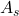= 40 % ; if we consider the width of the steel to be =40%;

Then , the concrete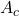= 60%

Moduli of concrete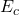= 50 GPa

Moduli of steel wire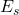= 100 GPa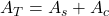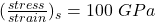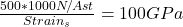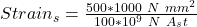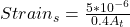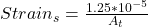Since;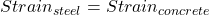Then: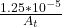=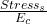Stress in the concrete =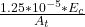Stress in the concrete =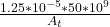Stress in the concrete =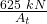However,0.6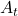=∴ Force transferred to  the concrete =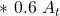Force  transferred to the concrete = 375 kN